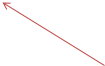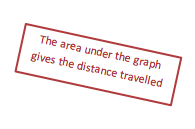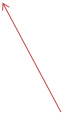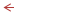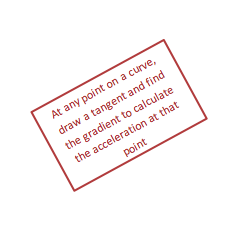# Equations of Motion

Use of suvat to solve problems

Defining Motion

·      Displacement, s (m) is the vector equivalent of distance – it is distance moved in a certain direction

·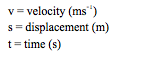Velocity, v (ms-1) is the vector equivalent of speed – it is the speed in a given direction

·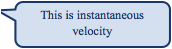Another way of describing this is to think of velocity as the rate of change of displacement:

·The rate of change of velocity is called acceleration – this is also a vector:

·      This equation assumes that acceleration is constant or uniform

Equations of Motion (suvat)

·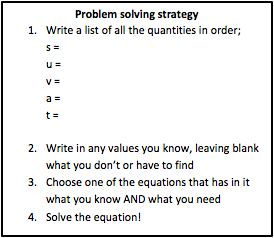These simple equations can be used to derive four equations that allow any problem involving an object moving under constant acceleration to be solved. They are given on your data sheet but it is best if you learn them:

Vertical and Horizontal Components of Velocity

·      Because velocity is a vector, like force, we can take horizontal and vertical components in the same way

·      Horizontal and vertical components of velocity are independent of each other

·      You can use the equations of motion with the horizontal or vertical components of velocity

·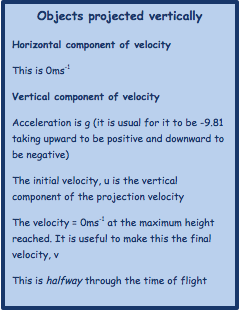You are expected to deal with 2 types of problem at AS level:Displacement-Time GraphsVelocity-Time Graphs